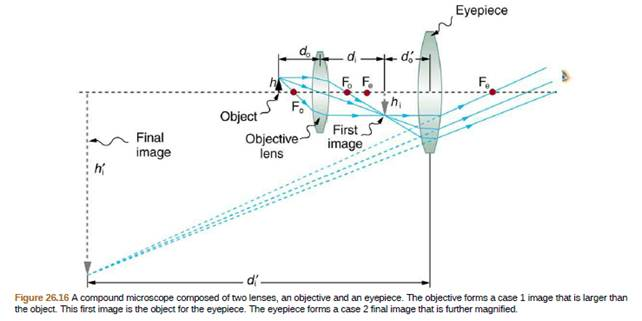Chapter 26, Problem 30PE

Chapter
Section
Textbook Problem

An amoeba is 0.305 cm away from the 0.300 cm focal length objective lens of a microscope. (a) Where is the image formed by the objective lens? (b) What is this image's magnification? (c) An eyepiece with a 2.00 cm focal length is placed 20.0 cm from the objective. Where is the final image? (c) What magnification is produced by the eyepiece? (e) What is the overall magnification? (See Figure 26.16.)To determine

(a)

Where is the image formed by the objective lens?

Explanation

Given:

Formula used:

The image distance of objective can be obtained using:

1fo=1do+1di      (1)

Isolating d i gets:

To determine

(b)

What is this image's magnification?

To determine

(c)

Where is the eyepiece final image?

To determine

(d)

What magnification is produced by the eyepiece?

To determine

(e)

What is the overall magnification?

Still sussing out bartleby?

Check out a sample textbook solution.

See a sample solution

The Solution to Your Study Problems

Bartleby provides explanations to thousands of textbook problems written by our experts, many with advanced degrees!

Get Started

Find more solutions based on key concepts